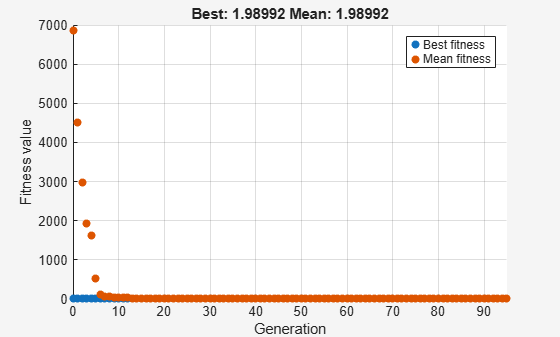# Minimize Rastrigins' Function Using `ga`, Problem-Based

This example shows how to minimize a function with multiple minima using the genetic algorithm in the problem-based approach. For two variables `x` and `y`, Rastrigin's function is defined as follows.

`ras = @(x, y) 20 + x.^2 + y.^2 - 10*(cos(2*pi*x) + cos(2*pi*y));`

Plot the function scaled by 10 in each direction.

```rf3 = @(x, y) ras(x/10, y/10); fsurf(rf3,[-30 30],"ShowContours","on") title("rastriginsfcn([x/10,y/10])") xlabel("x") ylabel("y")```The function has many local minima and a global minimum value of 0 that is attained at `x` = 0, `y` = 0. See What Is Global Optimization?

Create optimization variables `x` and `y`. Specify that the variables are bounded by $±100$.

```x = optimvar("x","LowerBound",-100,"UpperBound",100); y = optimvar("y","LowerBound",-100,"UpperBound",100);```

Create an optimization problem with the objective function `rastriginsfcn(x)`.

`prob = optimproblem("Objective",ras(x,y));`

Note: If you have a nonlinear function that is not composed of polynomials, rational expressions, and elementary functions such as `exp`, then convert the function to an optimization expression by using `fcn2optimexpr`. See Convert Nonlinear Function to Optimization Expression and Supported Operations for Optimization Variables and Expressions.

Create `ga` options to use the `gaplotbestf` plot function.

`options = optimoptions("ga","PlotFcn","gaplotbestf");`

Solve the problem using `ga` as the solver.

```rng default % For reproducibility [sol,fval] = solve(prob,"Solver","ga","Options",options)```
```Solving problem using ga. Optimization terminated: average change in the fitness value less than options.FunctionTolerance. ``````sol = struct with fields: x: 0.9950 y: 0.9950 ```
```fval = 1.9899 ```

Is the resulting function value the lowest minimum? Perform the search again. Because `ga` is a stochastic algorithm, the results can differ.

`[sol2,fval2] = solve(prob,"Solver","ga","Options",options)`
```Solving problem using ga. Optimization terminated: average change in the fitness value less than options.FunctionTolerance. ``````sol2 = struct with fields: x: 0.9950 y: -4.9289e-06 ```
```fval2 = 0.9950 ```

The second solution is better because it has a lower function value. A solution returned by `ga` is not guaranteed to be a global solution.